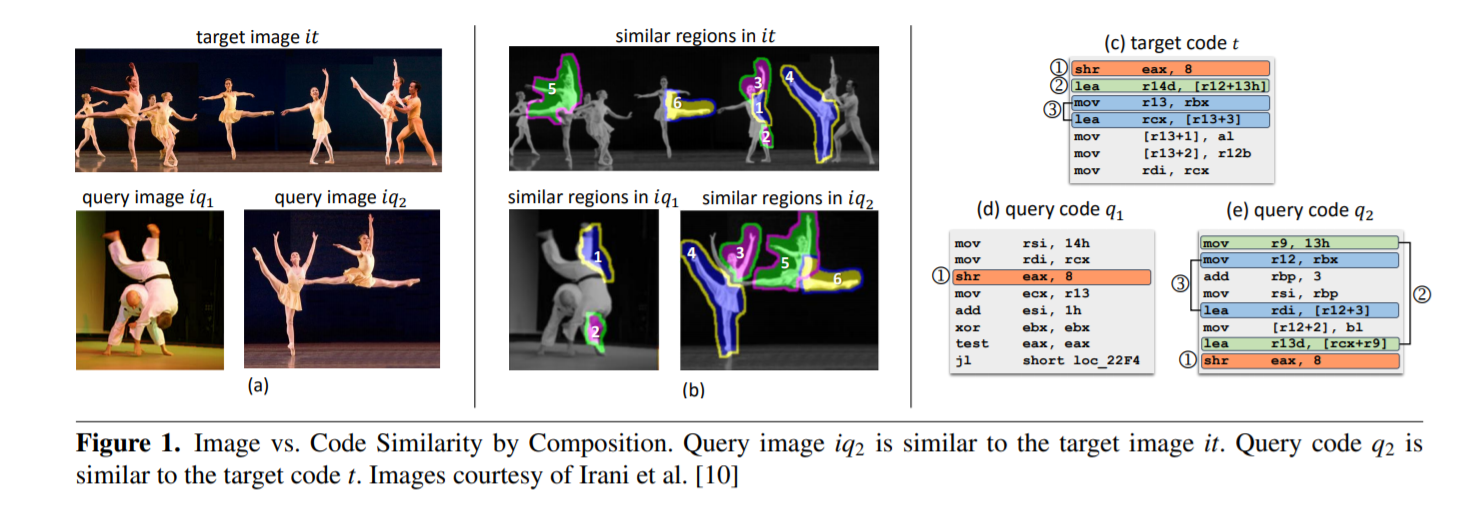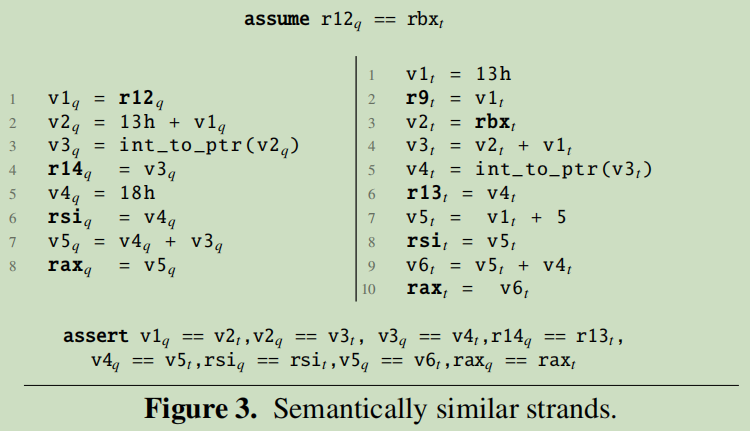# Statistical Similarity of Binaries阅读笔记

## 二进制代码相似性检测

Posted by Yunlongs on April 19, 2020

## Statistical Similarity of Binaries

github https://github.com/tech-srl/esh

### 前言

Similarity by composition： 如果一幅图像可以用另一幅图像的区域组成，那么它就相似于另一幅图像，并且这种相似性可以通过统计推理来量化。Intuition: 例如图1(a)展示了两个query image和一个target image，我们直觉上相信$iq_2$与$it$更为相似。现在我们将query images于target image相似的部分给用序号标记出来，如图1(b)所示，$iq_2$的大部分可以用$it$中图像片段合成出来，而$iq_1$只有小部分，所以我们认为$iq_2$与$it$更为相似。

### 方法概述Decomposition into strands: 将程序分解为strand作为基本单元来进行比较，再将strand转换为 Intermediatre Verification Language(IVL)，为每个strand的变量添加$q$和$t$后缀来标识不同的strand。

Comparing a pair of strands: 分为三步：

1. 为两个strands的输入增加相等性假设
2. 增加断言来检查所有输出变量的相等性。
3. 使用程序验证器来检查断言，并统计有多少变量是相等的

Match Probability: : 定义$s_q$与$s_t$之间的相似性为$s_q$中的变量在$s_t$中具有相等变量的百分比，用$VCP(s_q,s_t)$标识，但注意不对称。比如图3的例子，$VCP(s_q,s_t)=1$，而$VCP(s_t,s_q)=8/9$。

Local Evidence of Similarity: 定义$Pr(s_q|H_0)$为随机为$s_q$寻找一个匹配的概率（$H_0$表示所有可能的strands）。在$t$中为$s_q$寻找的匹配的重要性程度可以被定义为： $L E S\left(s_{q} | t\right)=\log \frac{\max _ {s_{s} \in \operatorname{Pr}\left(s_{q} | s_{t}\right)}}{\operatorname{Pr}\left(s_{q} | H_{0}\right)}$

Lifting strand similarity into procedure similarity: 两个程序$q$和$t$之间的global evidence of similarity(GES)就是在$q$中对所有的$s_q$的$LES(s_q|t)$进行累加。

### 3.Strand-Based Similarity by Composition

#### 3.2 Procedure Decomposition to Strands

1. 刚开始将block中所有指令全部加入unusedInsts
2. unusedInsts中取出最后一条指令为maxUsed
3. maxUsed开始向前遍历每一条指令，若此指令所定义的变量与maxUsed所引用的变量相同，则加入strand
4. 不断的扩充VarRefed和VarDefed，直到处理完所有指令

#### 3.3 Statistical Evidence

##### 3.3.1 Strand Similarity as a Probability Measure

$\operatorname{Pr}\left(s_{q} | t\right) \triangleq \max _{s_{i} \in H_{t}} \operatorname{Pr}\left(s_{q} | s_{t}\right) \tag 2$

$\operatorname{Pr}\left(s_{q} | s_{t}\right) \triangleq g\left(\operatorname{VCP}\left(s_{q}, s_{t}\right)\right)=1 /\left(1+e^{-k\left(V C P\left(s_{q}, s_{t}\right)-0.5\right)}\right) \tag 3$

##### 3.3.2 The Statistical Significance of a Strand

$\operatorname{Pr}\left(s_{q} | H_{0}\right)=\frac{\sum_{s_{t} \in T} \operatorname{Pr}\left(s_{q} | s_{t}\right)}{|T|}$

#### 3.4 Local and Global Evidence Scores

$L E S\left(s_{q} | t\right)=\log L R\left(s_{q} | t\right)=\log \operatorname{Pr}\left(s_{q} | t\right)-\log \operatorname{Pr}\left(s_{q} | H_{0}\right) \tag 5$

LES分数反应了$s_q$在$t$中具有非平凡语义相同strand的可行度等级，而GES分数反应了$q$可以由$t$中的一些非平凡部分组成的可行度等级。

#### 4.1 Similarity Semantics

preliminaries 定义程序状态$\sigma$为一个pair $(l,values)$，在一个确切的程序位置$l \in Loc$上将程序变量的集合映射到其具体的值:$Var \to Val$。 一个程序$P$所有可能状态的集合为$\Sigma_P$ 。程序的trace $\pi \in \Sigma_{P}^*$用来描述程序单个执行的状态序列$<\sigma_0,…,\sigma_n>$。程序$P$的所有可能traces标记为$[[P]]$。我们还定义$first$和$last$来返回trace中第一个和最后一个状态。

Variable correspondence： 两个状态$\sigma_1$,$\sigma_2$间的变量一致性表示为$\gamma:Var_1 \mapsto Var_2$，是从$\sigma_1$到$\sigma_2$中变量的有偏函数。$\Gamma(P_1,P_2)$为程序$(P_1,P_2)$对的所有变量一致性集合。

stata,trace equivalence: 给定两个状态和一个一致性$\gamma$，如果$\forall (v_1,v_2)\in \gamma:\sigma_1(v_1)=\sigma_2(v_2)$，那么我们就可以说对于$\gamma$这些状态是等价的，并且标记为$\sigma_1 \equiv_\gamma \sigma_2$。如果两个traces $last(\pi_1) \equiv_\gamma last(\pi_2)$，我们就成这些traces对于$\gamma$等价的，标记为$\pi_1 \equiv_\gamma \pi_2$。

(i) 在$\gamma$下，每一个来自$s_1$的输入都能与一些来自于$s_2$的输入匹配上。
(ii) 每一对traces $(\pi_1,\pi_2) \in (s_1,s_2)$ 在输入相同的情况下是等价的，即$\pi_1 \equiv_\gamma \pi_2$。